# ADDITIONAL INFORMATION TABLE 1 IS THE EXPERIMENT DATA. THE BOLT, THE DIMENSION OF THE BOLT. USING...

TABLE 1 IS THE EXPERIMENT DATA. THE BOLT, THE DIMENSION OF THE BOLT. USING THE INFORMATION FROM TABLE 1 I'M SUPPOSED TO MAKE ANOTHER TABLE SHOWING make another table that reports tu, ty,tys both calculated from the data in table 1 using the equation from number 5 (torsional yield strength) and another column with the estimated calculations using section 7 equation and getting the information(torsional yield strength numbers from the bolt chart for each specimen)

Next in the table find tus (Calculated from the data using # 6 equation (ULTIMATE TORSIONAL STRENGTH below then and an estimated calculation using #8 equation ultimate torsional strength using the information in the bolt chart). All the dimensions and information from table 1 is to make a second table 2 with the information above.

_______________________________________________________________________________________________

my calculations in table 2 are not correct i need to redo Table 2.

what is wrong with my data see instructions in photo 2 second bullett point -------

make another table that reports Tu, Ty,tys (calculated from the data using section 5 and estimated using section 7) and tus (Calculator from the data using section 6 and estimated using section 8)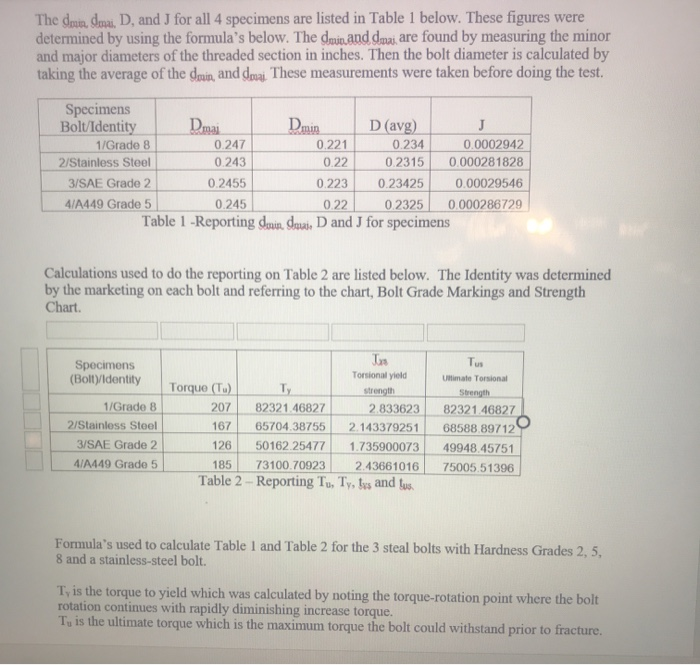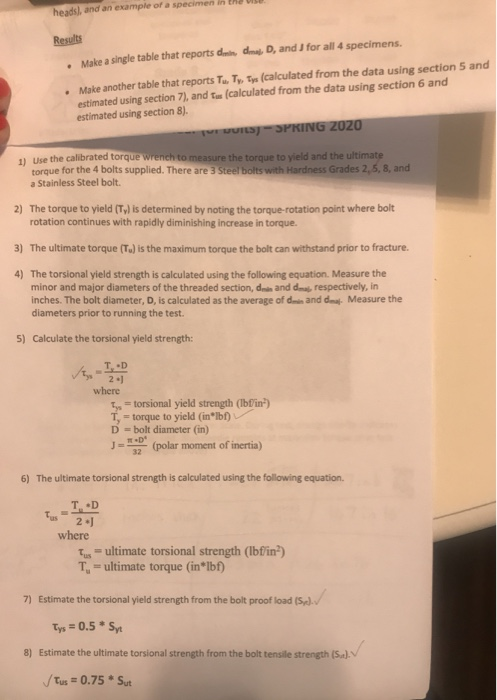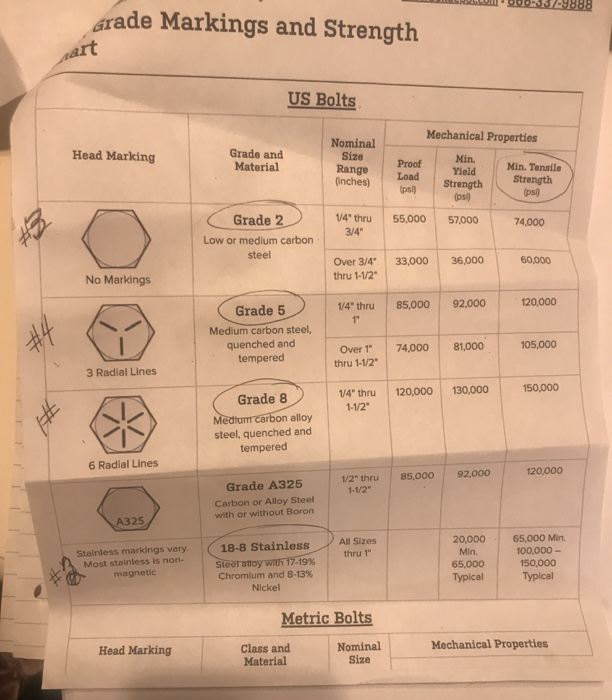The doua mi. D, and J for all 4 specimens are listed in Table 1 below. These figures were determined by using the formula's below. The demand daai are found by measuring the minor and major diameters of the threaded section in inches. Then the bolt diameter is calculated by taking the average of the drain and doi These measurements were taken before doing the test. Dmin Specimens Bolt/Identity Dmi D (avg) 1/Grade 8 0.247 0.221 0.234 0.0002942 2/Stainless Steel 0.243 0.22 0.2315 0.000281828 3/SAE Grade 2 0.2455 0.223 0.23425 0.00029546 4/A449 Grade 5 0.245 0.2325 0.000286729 Table 1 - Reporting deuindou. D and J for specimens Calculations used to do the reporting on Table 2 are listed below. The Identity was determined by the marketing on each bolt and referring to the chart, Bolt Grade Markings and Strength Chart. Tus Specimens (Bolt)/identity 1/Grade 8 2/Stainless Steel 3/SAE Grade 2 4/A449 Grade 5 Torsional yield Torque (T.) strength 207 82321.46827 2.833623 167 65704.38755 2.143379251 126 50162 25477 1.735900073 185 73100.70923 2.43661016 Table 2 - Reporting T., Ty, tus and tus Ultimate Torsional Strength 82321.46827 68588 89712 49948.45751 75005,51396 Formula's used to calculate Table 1 and Table 2 for the 3 steal bolts with Hardness Grades 2, 5, 8 and a stainless steel bolt. T, is the torque to yield which was calculated by noting the torque-rotation point where the bolt rotation continues with rapidly diminishing increase torque. T, is the ultimate torque which is the maximum torque the bolt could withstand prior to fracture.
heads, and an example of a specimen in the VILE Results • Make a single table that reports didma, D, and for all 4 specimens. • Make another table that reports T. Ty Tys (calculated from the data using section 5 and estimated using section 7), and us (calculated from the data using section 6 and estimated using section 8). EUILS - SPRING 2020 11 Use the calibrated torque Wrench to measure the torque to yield and the ultimate torque for the 4 bolts supplied. There are 3 Steel bolts with Hardness Grades 2,5,8, and a Stainless Steel bolt. 2) The torque to yield (Ty) is determined by noting the torque-rotation point where bolt rotation continues with rapidly diminishing increase in torque. 3) The ultimate torque (T) is the maximum torque the bolt can withstand prior to fracture. 4) The torsional yield strength is calculated using the following equation. Measure the minor and major diameters of the threaded section, die and des respectively, in inches. The bolt diameter, D, is calculated as the average of dmin and mat. Measure the diameters prior to running the test. 5) Calculate the torsional yield strength: where Iyo torsional yield strength (lbf'in) T-torque to yield (inⓇlbf) D = bolt diameter (in) J = *** (polar moment of inertia) 6) The ultimate torsional strength is calculated using the following equation where Tus = ultimate torsional strength (lbfin) T. - ultimate torque (in*lbf) 7) Estimate the torsional yield strength from the bolt proof load (5.). Tys = 0.5 * Syt 8) Estimate the ultimate torsional strength from the bolt tensile strength (S.). V tus = 0.75 * Sut
Grade Markings and Strength US Bolts Mechanical Properties Head Marking Grade and Material Nominal Size Range (inches) Proof Load ipsi) Min. Yield Strength (psi) Min. Tensile Strength (psi 14" thru 3/4" 55,000 57.000 Grade 2 Low or medium carbon steel 74,000 33,000 36,000 Over 3/4" thru 1-1/2 60,000 No Markings 1/4" thru 85,000 92,000 120,000 (Grade 5 Medium carbon steel, quenched and tempered 74,000 81,000 105,000 Over 1" thru 1-1/2 3 Radial Lines 1/4" thru 120,000 130,000 150,000 1-1/2" Grade 8 Medium carbon alloy steel, quenched and tempered 6 Radial Lines 85.000 92,000 120,000 1/2" thru 1-1/2" Grade A325 Carbon or Alloy Steel with or without Boron A325 All Sizes thru 1" Stainless markings vary. Most stainless is non- magnetic (18-8 Stainless Steerattay with 17-19% Chromlum and 8-13% Nickel 20,000 Min. 65,000 65,000 Min. 100,000 150,000 Typical X Typical Metric Bolts Head Marking Class and Material Nominal Size Mechanical Properties

The corrected table-2 is hereunder:

 Specimens Bolt/Identity Ultimate Torque (Tu) Yield Torque (Ty) Torsional Yield Strength (Tys) Ultimate Torsional Strength (Tus) Tys=Ty*D/2J Tys=0.5*Syt Tus=Tu*D/2*J Tus=0.75*Sut 1/Grade 8 207 82321.46827 32.73831×10^6 60,000 82.32155×10^3 112,500 2/Stainless Steel 167 65704.38755 26.98554×10^6 68.58882×10^3 48,750 3/SAE Grade 2 126 50162.25477 19.88511×10^6 27,500 49.94838×10^3 55,500 4/A449 Grade 5 185 73100.70923 29.63759×10^6 42,500 75.00549×10^3 90,000

#### Earn Coin

Coins can be redeemed for fabulous gifts.

Similar Homework Help Questions
• ### Please show solution for O step by step, use data and ansers for N as given (O)For the bolt of question (N) above, what is the required tightening torque? Answer Box: Torque 141 lb-in Total Clam...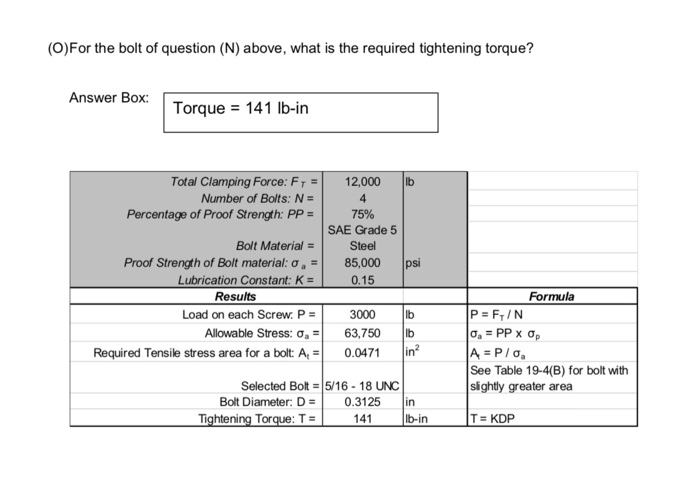Please show solution for O step by step, use data and ansers for N as given (O)For the bolt of question (N) above, what is the required tightening torque? Answer Box: Torque 141 lb-in Total Clamping Force: F,-| 12,000 |lb Number of Bolts: N- Percentage of Proof Strength: PP- 75% SAE Grade 5 Steel Bolt Material Proof Strength of Bolt material: σ a 85,000 psi Lubrication Constant: K 0.15 Results Formula P FrIN Load on each Screw. P- 3000 b...

• ### Given: Section view of a bolt circle, Sut -120Kpsi Each bolt is loaded by a repeating force of 0 ...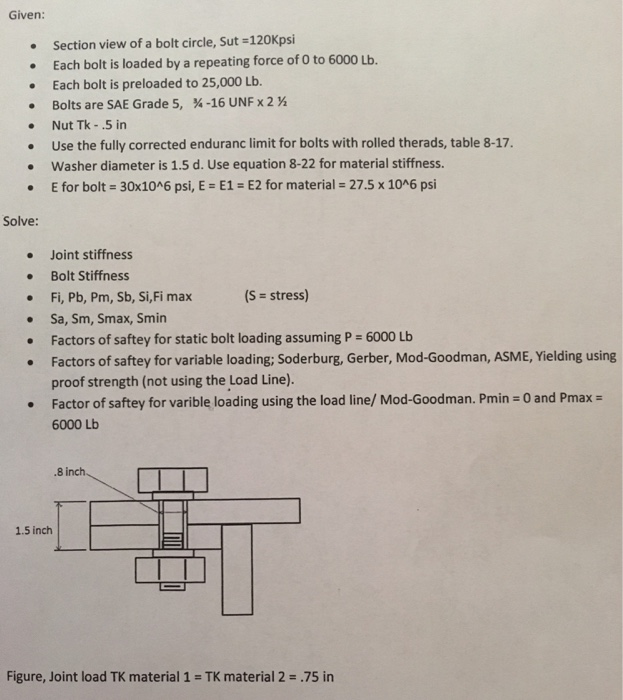Given: Section view of a bolt circle, Sut -120Kpsi Each bolt is loaded by a repeating force of 0 to 6000 Lb Each bolt is preloaded to 25,000 Lb. Bolts are SAE Grade 5, -16 UNF x 2 Nut Tk -.5 in . Use the fully corrected enduranc limit for bolts with rolled therads, table 8-17. . Washer diameter is 1.5 d. Use equation 8-22 for material stiffness. . E for bolt- 30x1046 psi, E E1- E2 for material 27.5...

• ### 5. Using AISC Steel Construction Manual, find the following design strengths of 1-inch Group A bolts on A36 gusset plates, type N, with STD holes, 3" bolt spacing and 2" edge distance: (5...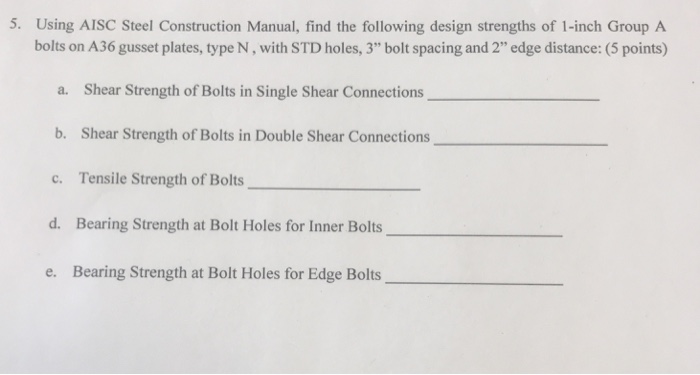5. Using AISC Steel Construction Manual, find the following design strengths of 1-inch Group A bolts on A36 gusset plates, type N, with STD holes, 3" bolt spacing and 2" edge distance: (5 points) a. Shear Strength of Bolts in Single Shear Connections b. Shear Strength of Bolts in Double Shear Connections c. Tensile Strength of Bolts d. Bearing Strength at Bolt Holes for Inner Bolts e. Bearing Strength at Bolt Holes for Edge Bolts 5. Using AISC Steel Construction...

• ### True of False 1.If a material point has three principal stresses ?1 = ?2 = ?3...

True of False 1.If a material point has three principal stresses ?1 = ?2 = ?3 = 300????, then the maximum shear stress is ???? = 150????. 2.The endurance limit of a material is independent on the size of a part. 3.The tensile stress in a bolt is usually calculated through tensile force divided by area of unthreaded rod’s cross-section area. 4.Edge shear-out or tear-out is one failure mode in bolted or riveted joints. 5.Pretension of bolts can be controlled...

• ### Question 1: Three tension members are shown below. Assume the strength of the member controls and...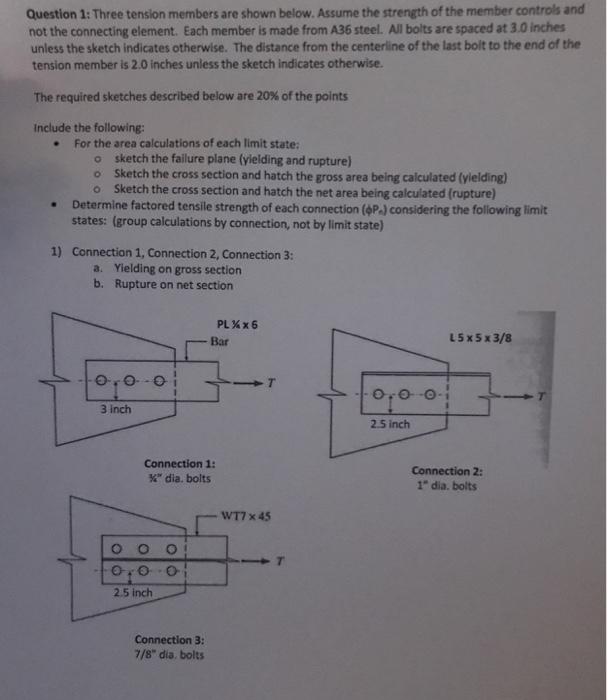Question 1: Three tension members are shown below. Assume the strength of the member controls and not the connecting element. Each member is made from A36 steel. All bolts are spaced at 3.0 inches unless the sketch indicates otherwise. The distance from the centerline of the last bolt to the end of the tension member is 2.0 inches unless the sketch indicates otherwise. The required sketches described below are 20% of the points Include the following: For the area calculations...

• ### 1. By comparing your researched values, obtained through the experiment, with those of the steel manufacturer's:...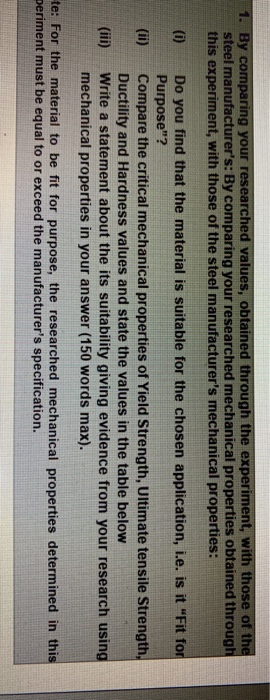1. By comparing your researched values, obtained through the experiment, with those of the steel manufacturer's: By comparing your researched mechanical properties obtained through this experiment, with those of the steel manufacturer's mechanical properties: (i) Do you find that the material is suitable for the chosen application, i.e. is it "Fit for Purpose"? Compare the critical mechanical properties of Yield Strength, Ultimate tensile Strength, Ductility and Hardness values and state the values in the table below Write a statement about...

• ### 1) Using ultimate stress values from textbook table 21.3, a factor of safety of 1.5 and...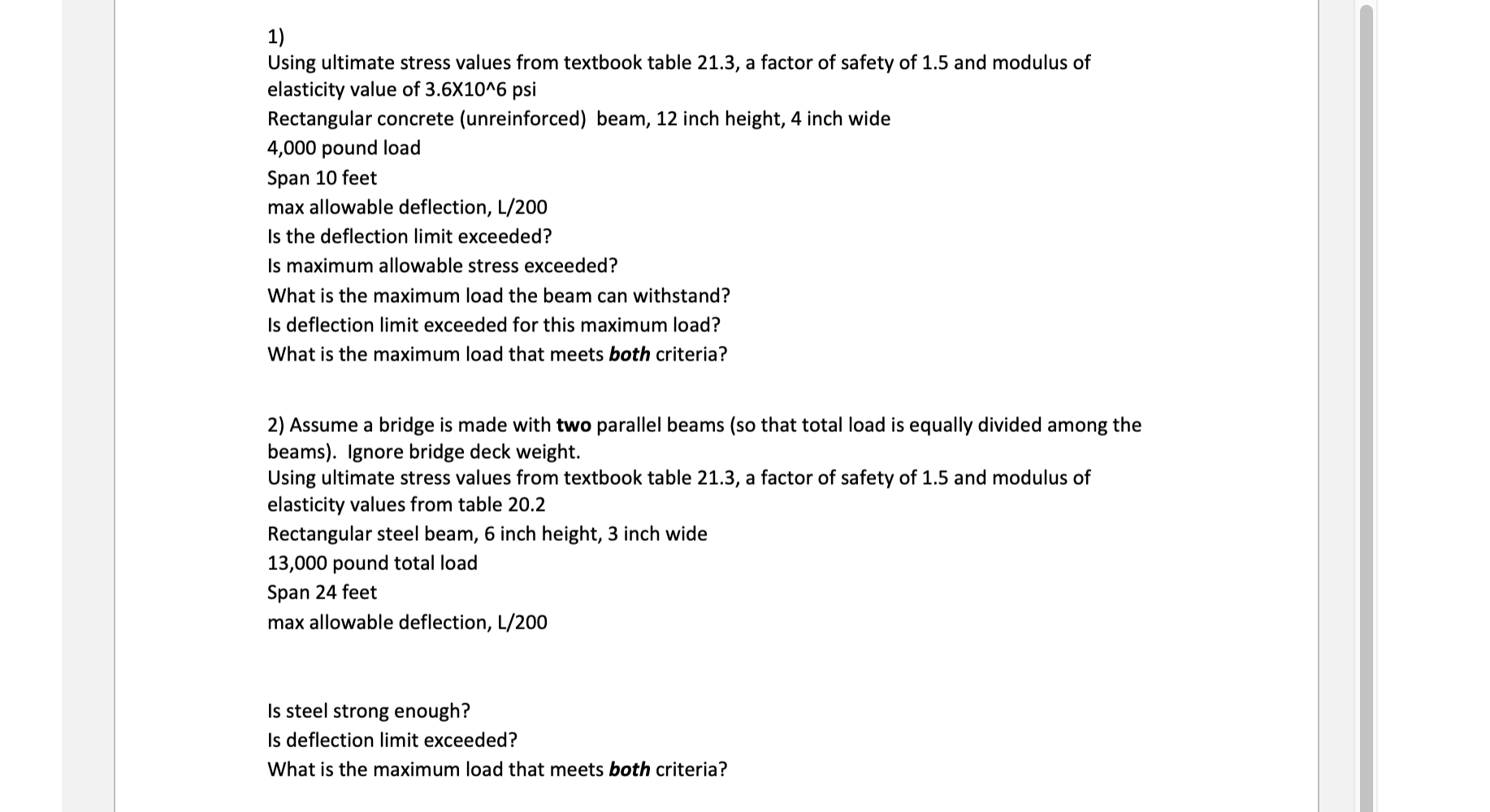1) Using ultimate stress values from textbook table 21.3, a factor of safety of 1.5 and modulus of elasticity value of 3.6X10^6 psi Rectangular concrete (unreinforced) beam, 12 inch height, 4 inch wide 4,000 pound load Span 10 feet max allowable deflection, L/200 Is the deflection limit exceeded? Is maximum allowable stress exceeded? What is the maximum load the beam can withstand? Is deflection limit exceeded for this maximum load? What is the maximum load that meets both criteria? 2)...

• ### 1. Complete the table below for the welded connection samples shown. ETNICES Ful enetration But Weld Partial Penet...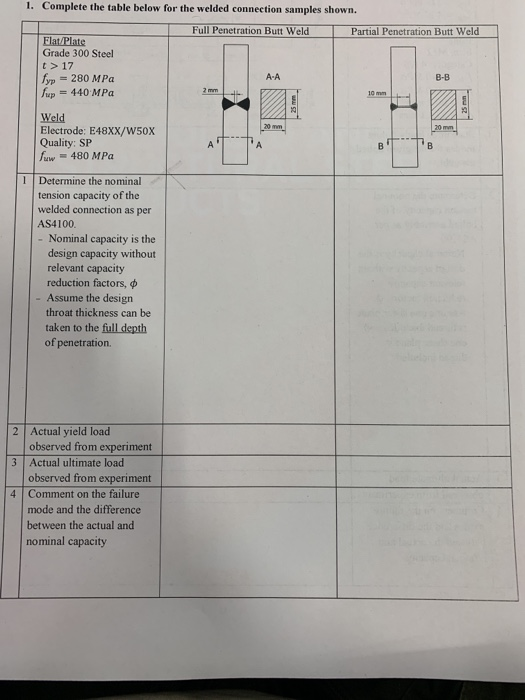1. Complete the table below for the welded connection samples shown. ETNICES Ful enetration But Weld Partial Penetration Burt Weld Flat/Plate Grade 300 Steel t>17 fyp 280 MPa up 440 MPa A-A B-B 10mm Weld Electrode: E48XX/W50X Quality: SP w480 MPa 1 Determine the nominal tension capacity of the welded connection as per AS4100 Nominal capacity is the design capacity without relevant capacity reduction factors Assume the design throat thickness can be taken to the full depth of penetration. 2...

• ### 3. Tentukan rintangan tiang terbina dengan keratan-rentas yang ditunjukkan dalam Rajah 3 di bawah mampatan tulen....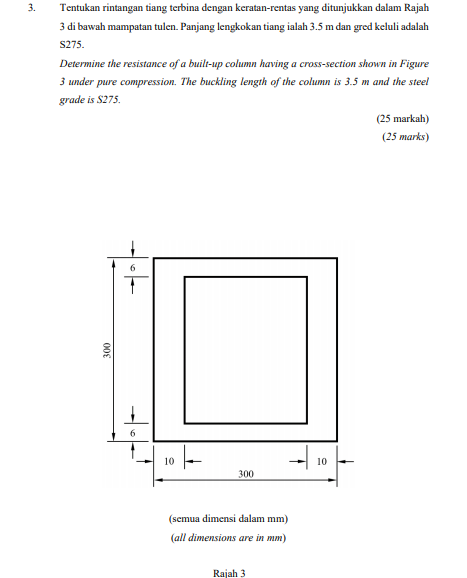3. Tentukan rintangan tiang terbina dengan keratan-rentas yang ditunjukkan dalam Rajah 3 di bawah mampatan tulen. Panjang lengkakan tiang ialah 3.5 m dan gred keluli adalah S275. Determine the resistance of a built-up column having a cross-section shown in Figure 3 under pure compression. The buckling length of the column is 3.5 m and the steel grade is \$275. (25 markah) (25 marks) 701 300 6 10 1 10 300 (semua dimensi dalam mm) (all dimensions are in mm) Rajah...

• ### 1. The gears have full depth teeth and are manufactured from AISI 1020 CD (Through hardened...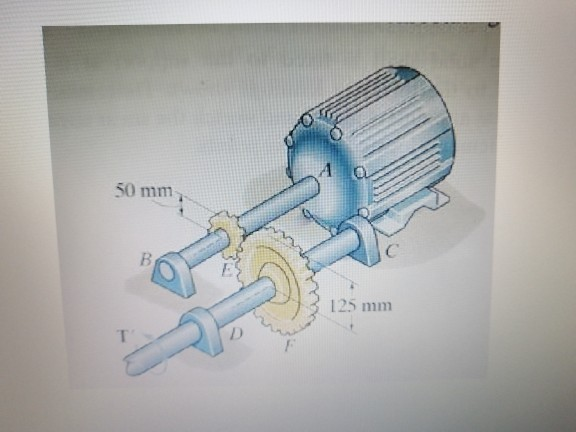1. The gears have full depth teeth and are manufactured from AISI 1020 CD (Through hardened grade 1 steel) to 248 BHN and have a module of 3 mm/tooth with a pressure angle of 20 degrees. The motor develops a peak power of 44.54 kW at 1,869 rpm. Use a factor of safety of 8 from the yield strength for the shaft. The shaft is made from AISI 1040 CD steel. Estimate the maximum bending moment in shaft CD. Give...